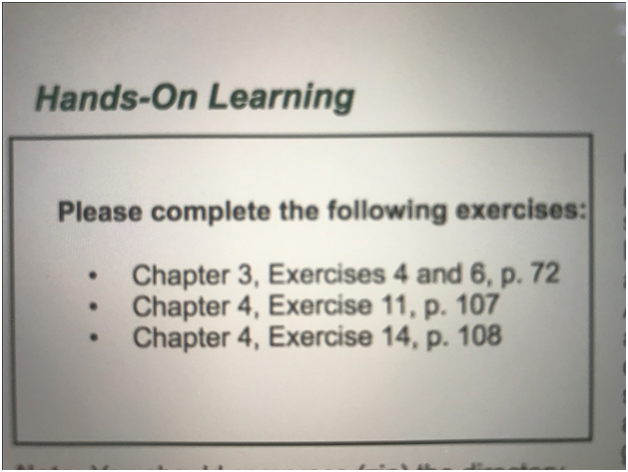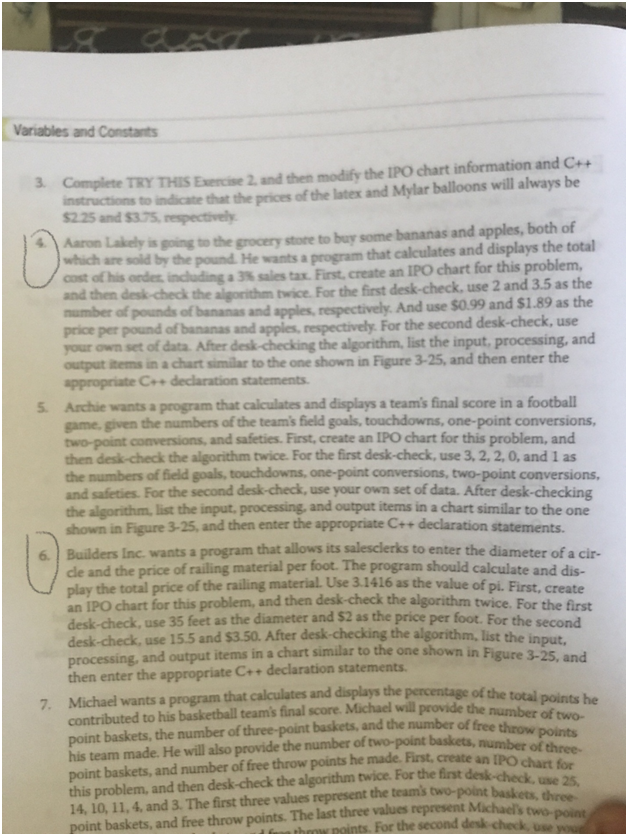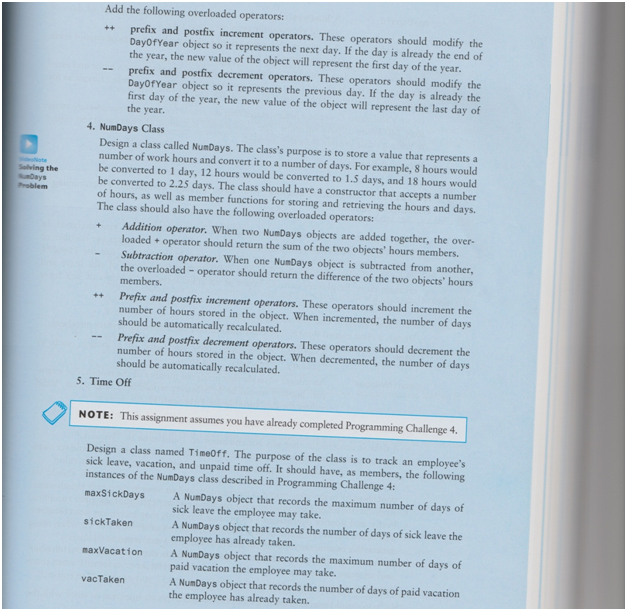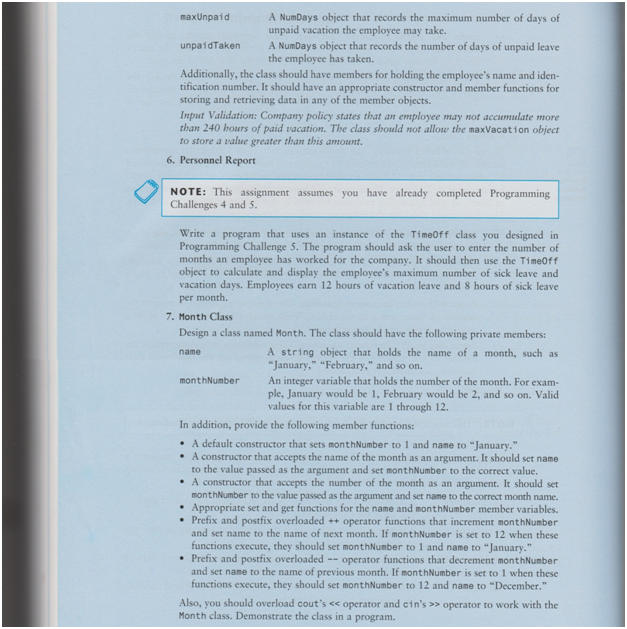Do you wish to have someone answer your programming assignment questions on your behalf? You are at the right place. We have more than 300 programming experts working round the clock to offer the academic support you need and deserve.

## 4 quick questionsSolution

### Exercise 4

Table 1: IPO chart for the problem
 Input Processing Output ·         Aaron Lakely's order of buying bananas and apples ·         unit price of banana and apple Processing Items: ·         banana amount ·         apple amount ·         banana unit price ·         apple unit price ·         tax rate   Algorithm: 1.       Input banana amount and apple amount 2.       Input banana unit price and apple unit price 3.       Calculate product cost: product cost = banana*banana unit price + apple * apple unit price 4.       Calculate total cost: total cost = product cost + (product cost * 0.03) 5.       Display total cost Total cost on the screen
Desk-Check Algorithm
1. number of punds of banana = 2, number of pounds of apple = 3.5, unit price of banana = 0.99\$, unit price of apple = 1.89\$
 Step Banana pounds Apple pounds Banana unit price Apple unit price Product cost Total cost Input/Output 1 2 3.5 B=2 A=3.5 2 0.99 1.89 Bp=0.99 Ap=1.89 3 pcost=2*0.99+3.5*1.89=10.3572 4 tcost=10.3572+10.3572*0.03=10.667916 5 total=10.67
1. my own set: number of punds of banana = 5, number of pounds of apple = 7, unit price of banana = 1\$, unit price of apple = 3\$
 Step Banana pounds Apple pounds Banana unit price Apple unit price Product cost Total cost Input/Output 1 5 7 B=5 A=7 2 1 3 Bp=1 Ap=3 3 pcost=5*1+7*3=26 4 tcost=26+26*0.03=26.78 5 total=26.78

## C++ statements

// init

float B, A, Bp, Ap;

float pcost, tcost;

// step 1

cout<<“B=”; cin>>B;

cout<<“A=”; cin>>A;

// step 2

cout<<“Bp=”; cin>>Bp;

cout<<“Ap=”; cin>>Ap;

//step 3

pcost = B * Bp + A * Ap;

// step 4

tcost = pcost + pcost * 0.03;

// step 5

cout<<“total=”<

### Exercise 6

Table 2: IPO chart for this problem
 Input Processing Output ·         diameter of circle ·         price of railing material per foot Processing Items: ·         diameter of circle ·         price of railing material per foot ·         PI number   Algorithm: 1.       Input diameter of circle 2.       Input unit price 3.       Calculate perimeter of circle: perimeter of circle=diameter of circle*PI number 4.       Calculate cost: cost=perimeter of circle*unit price 5.       Display cost Display the cost on the screen
Desk-Check Algorithm
1. diameter of circle = 35, price of railing material per foot = 2\$
 Step Diameter of circle Unit price Perimeter of circle Cost Input/Output 1 35 D=35 2 2 unit per foot=2 3 perimeter=35*3.1416=109.956 4 cost=109.956*2=219.912 5 Cost=219.912
1. diameter of circle = 345, price of railing material per foot = 5.78\$
 Step Diameter of circle Unit price Perimeter of circle Cost Input/Output 1 345 D=345 2 5.78 unit per foot=5.78 3 perimeter=345*3.1416=1083.852 4 cost=1083.852*5.78=6264.66456 5 Cost=6264.66456

## C++ statements

// init

float D, upf, cost, perimeter, PI = 3.1416;

// step 1

cout<<“D=”; cin>>D;

// step 2

cout<<“unit per foot=”; cin>>upf;

// step 3

perimeter = D * PI;

// step 4

cost = perimeter + upf;

// step 5

cout<<“Cost=”<

Chapter 4

### Exercise 11

Table 3: IPO chart for this problem
Input ·         beginning balance ·         total deposits ·         total withdrawals   Processing Process Items ·         beginning balance ·         total deposits ·         total withdrawals Algorithm 1.       Input beginning balance, total deposits, total withdrawals 2.       Calculate account balance: balance=beginning blance+total deposits-total withdrawals 3.       Display current balance Output Display current balance on the screen

## C++ statements

// init float BB, TD, TW, balance; // step 1 cout<<"Beginning balance="; cin>>BB; cout<<"Total deposits="; cin>>TD; cout<<"Total withdrawals="; cin>>TW; // step 2 balance = BB + TD - TW; // step 3 cout<<"Balance="<
Full source code of Introductory11.cpp:
 #include using namespace std; int main() { // init float BB, TD, TW, balance; // step 1 cout<<"Beginning balance="; cin>>BB; cout<<"Total deposits="; cin>>TD; cout<<"Total withdrawals="; cin>>TW; // step 2 balance = BB + TD - TW; // step 3 cout<<"Balance="<
Desk-Check Algorithm & program
1. beginning balance=2545.75, total deposits=409.43, total withdrawals=210.65
 Step Beginning balance Total deposits Total withdrawals Balance Input/Output 1 2545.75 409.43 210.65 Beginning balance=2545.75 Total deposits=409.43 Total withdrawals=210.65 2 balance=2545.75+409.43-210.65=2744.53 3 Balance=2744.53
1. beginning balance=1125.33, total deposits=23, total withdrawals=800.94
 Step Beginning balance Total deposits Total withdrawals Balance Input/Output 1 1125.33 23 800.94 Beginning balance=1125.33 Total deposits=23 Total withdrawals=800.94 2 balance=1125.33+23-800.94=347.39 3 Balance=347.39

### Exercise 14

Table 4: IPO chart for this problem
Input ·         number of small size sold ·         number of medium size sold ·         number of large size sold ·         number of family size sold   Processing Process Items ·         number of small size sold ·         number of medium size sold ·         number of large size sold ·         number of family size sold Algorithm 1.       Input number of small size sold, number of medium size sold, number of large size sold, number of family size sold 2.       Calculate total number of pizza was sold: total=number of small size+number of medium size+number of large size+ number of family size 3.       Calculate percentage of each type: percent of small=100*number of small/total; percent of medium=100*number of medium/total; percent of large=100*number of large/total, percent of family= 100*number of family/total 4.       Display total sold and percentage of each type sold Output ·         Display total sold ·         Display small percent, medium percent, large percent, family percent

## C++ statements

#include using namespace std;   int main() { // init int small, medium, large, family, total; float small_percent, medium_percent, float large_percent, family_percent;   // step 1 cout<<"small="; cin>>small; cout<<"medium="; cin>>medium; cout<<"large="; cin>>large; cout<<"family="; cin>>family; // step 2 total = small + medium + large + family; // step 3 small_percent = 100.0 * small / total; medium_percent = 100.0 * medium / total; large_percent = 100.0 * large / total; family_percent = 100.0 * family / total; // step 4 cout<<"Total pizza sold="<
Step Small Medium Large Family Total Percentages Input/Output
1 25 50 50 75 small=25 medium=50 large=50 family=75
2 total=25+50+50+75=200
3 smallp=100*25/200=12.5 mediump=100*50/200=25 largep=100*50/200=25 familyp=100*75/200=37.5
4 Total pizza sold=200 Small percent=12.5 Medium percent=25.0 Large percent=25.0 Family percent=37.5
1. small=30, medium=25, large=85, family=73
 Step Small Medium Large Family Total Percentages Input/Output 1 30 25 85 73 small=30 medium=25 large=85 family=73 2 total=30+25+85+73=213 3 smallp=100*30/213=14.1 mediump=100*25/213=11.7 largep=100*85/213=39.9 familyp=100*73/213=34.3 4 Total pizza sold=213 Small percent=14.1 Medium percent=11.7 Large percent=39.9 Family percent=34.3